Density Of Matter

Density of Matter

Density is the quantity of mass in a unit of volume. It is the distinguishing property of matter. Each matter has its own density. Representation of density is d; unit of it is g/cm³.

Formula of density;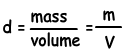We can show this relation with graphs also, look at the given graphs;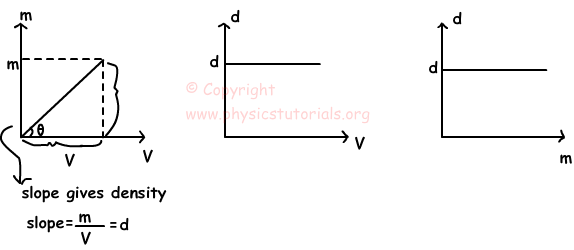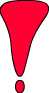Be careful!! These graphs show the relations of mass, density and volume under constant temperature. Changes in the temperature change the values of volume and density. As you can see from the graphs increase in the volume and mass does not affect the value of density, because it is constant under constant temperature and characteristic property of matter.

Example: Find the densities of the given matters;

a) Matter having mass 100g and volume 50cm³

b) Matter having 125g and volume 100cm³

a) Density=mass/volume=100g/50cm³=2g/cm³

b) Density=mass/volume=125g/100cm³=1,25g/cm³

Example: Find the density of the given matter using the graph below;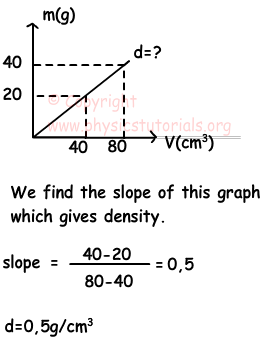Density of Mixtures

Mixtures include at least two different matters. If the matters are homogeneously mixed than we called them homogeneous mixtures, on the contrary if they do not mixed homogeneously we called them heterogeneous mixtures. Now we will learn how to calculate the density of homogeneous mixtures. Let me start with a mixture including different matters.

Matter 1: has mass m1 and volume V1

Matter 2: has mass m2 and Volume V2

Matter n: has mass mnand volume Vn

Density=mass/volume

If we have more than one substance;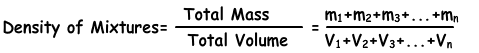Example: Find the density of the mixture including three different matters having mass 25g, 35g and 65g, and volumes 10cm³, 25cm³, and 50cm³.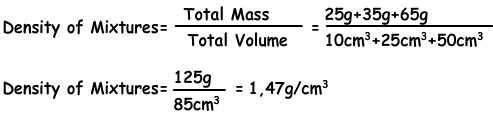Some Tricks for Special Cases in Density of Mixtures

• · If the volumes of the matters are equal in a given mixture then we calculate the density of mixture with following formula;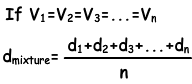• · If the masses of the matters are equal in a given mixture then we calculate the density of the mixture with following formula;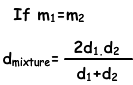Example: The graph given below shows the relation of masses vs. volumes of two different matters A and B. If we take 40cm³ from A and 40cm³ from B volume, calculate the density of the mixture.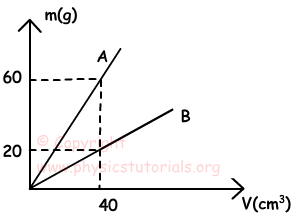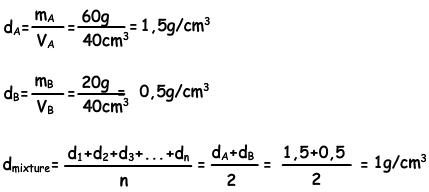Author: